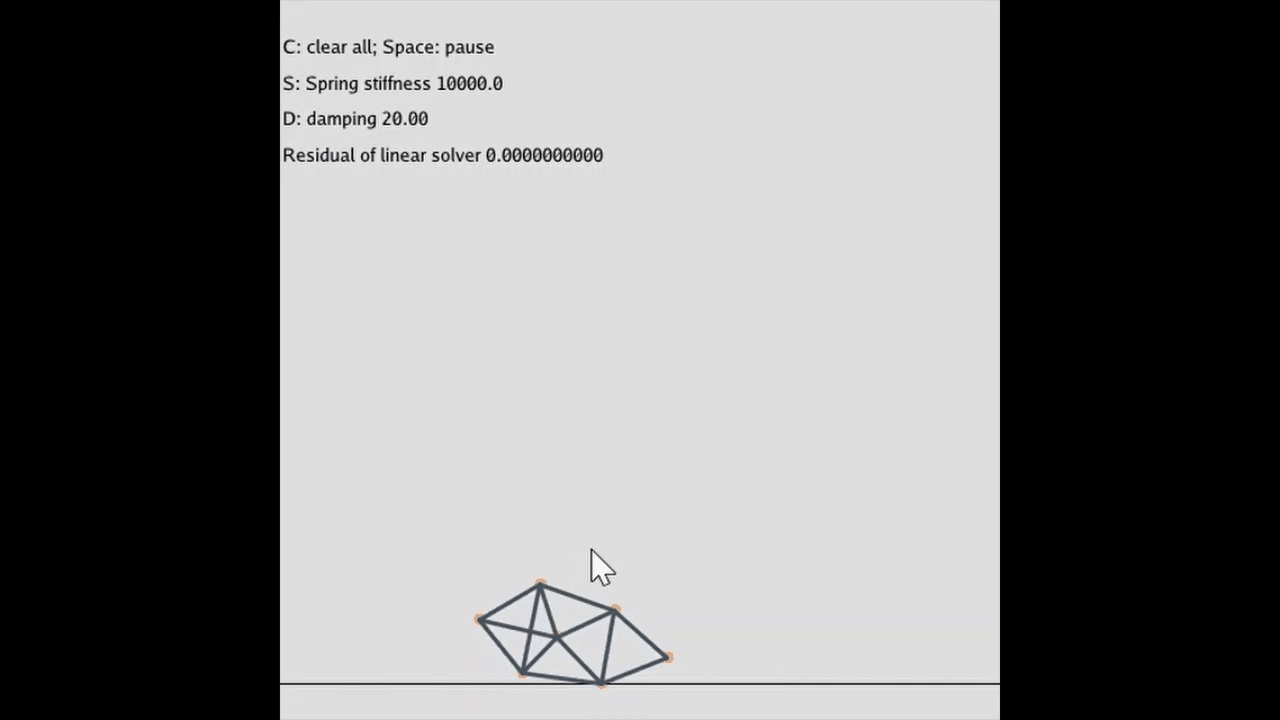# Physical Simulation：Mass-spring systems质点弹簧系统是一种拉格朗日视角的物理模拟，把物体看成由一个个质点构成，质点之间通过弹簧相连，从而产生一定程度的弹性形变。质点弹簧系统适用于模拟布料软体、刚体等物理材质。

## 一、胡克定律

对于物体中的任意两个不同的质点$x_i$和$x_j$，从$j$作用到$i$的作用力可由以下的胡克定律（Hooke’s law）给出：

其中$k$是弹簧刚度系数（spring sitffness），$l_{ij}$是质点之间的弹簧静止长度，$\widehat{\mathbf{x_i}-\mathbf{x_j}}$是从质点$i$指向质点$j$的单位方向向量。对于质点$x_i$，它收到的作用力就是与之相连的所有弹性力的合力：

求出了合力，再根据牛顿第二定律可求出质点的加速度和位置的变化梯度：

上面就是质点弹簧系统的核心公式。

## 二、显式积分求解

一种最简单的求解方法就是采用前向欧拉积分（显式积分）：

但通常更常用的是下面的半隐式欧拉积分法（依旧是显式积分），区别在于更新$\mathbf{x_{t+1}}$是用$\mathbf{v_t}$还是$\mathbf{v_{t+1}}$：

使用半隐式欧拉法的求解步骤可以简单地分成如下的步骤：

• Compute new velocity using $\mathbf{v_{t+1}}= \mathbf{v_t}+\Delta t\frac{\mathbf{f_t}}{m}$

• Collision detection and handling

• Compute new position using $\mathbf{x_{t+1}}=\mathbf{x_t}+\Delta t \mathbf{v_{t+1}}$

显式积分的求解比较简单，不难理解，这里不再细说。下面的代码实现了显式积分求解的质点弹簧系统。

显式积分求解的优点就是简单方便、计算量小，但它也有致命的缺点，就是很容易导致模拟的数值爆炸现象。为了防止数值爆炸，显式积分器对模拟的时间步长有如下的严格要求：

可以看到时间步长的上限与物体的刚度系数$k$密切相关，刚度系数越大，则时间步长应该越小，否则将不满足上述条件而爆炸。因此显式积分器对于高刚度系数的物体不友好，稳定性不够。

## 三、隐式积分求解

隐式积分器从后向欧拉法展开，将原本显式积分的公式$(5)$换成下述形式：

其中$\mathbf{M}^{-1}$是对角矩阵，对角线上的元素取值为质点质量的倒数。通俗地讲就是未来的物理量依旧取决于未来的状态，乍一看是一个鸡生蛋还是蛋生鸡的死循环问题。我们先把上面的$\mathbf{x_{t+1}}$替换到下面的$\mathbf{v_{t+1}}$公式中，有：

然后对$\mathbf{f}(\mathbf{x_t}+\Delta t \mathbf{v_{t+1}})$做一阶泰勒展开可得：

再经过移项整理，就有如下的形式：

对于每一个质点，都有上述的公式$(8)$。公式$(8)$中左边是一个未知量$\mathbf{v_{t+1}}$乘上系数矩阵，右边是已知量，这是一个线性方程。把所有的质点的公式$(8)$组装起来，就得到了一个大规模的线性方程组。设质点数量为$n$，则左边的系数矩阵为$n\times n$的矩阵，每个矩阵的元素又是一个小矩阵（对于二维情况，就是$2\times 2$，而三维就是$3\times 3$），未知量向量长度为$n$，每个向量的元素也是一个小向量（对于二维情况，就是$2$，而三维就是$3$），右边同理：

所以隐式积分器是先求解出上述方程中的$\mathbf{v}_{t+1}$，然后再利用$\mathbf{v}_{t+1}$去更新质点的位置向量。上述公式中还有一个比较难搞的就是$\frac{\partial \mathbf{f}}{\partial \mathbf{x}}$项，$\mathbf{f}$是一个多元向量，$\mathbf{x}$亦是一个多维向量，因此$\mathbf{f}$关于$\mathbf{x}$是的求导结果是一个雅可比矩阵（二维情况下该矩阵大小为$2\times 2$，更高维依次类推）。关于雅可比矩阵，请看这个维基百科链接，这里不再赘述。

关于$\frac{\partial \mathbf{f}}{\partial \mathbf{x}}$的推导请看这里，最终的计算公式如下：

上面是关于$\mathbf{x}_i$的求导，而关于$\mathbf{x}_j$的求导则是取相反符号即可：

对于质点$\mathbf{x}_i$，它通常收到多个弹力，因此其最终的雅可比矩阵为多个导数的求和：

接下来就是如何求解线性方程组$\mathbf{A}\mathbf{v}_{t+1}= \mathbf{b}$的问题，一种简单、容易并行的方法就是雅可比迭代，关于雅可比迭代的具体细节请看这里。雅可比迭代与高斯消元法不同，它采用迭代过程渐进逼近地方式来求方程的解，虽然要有一定的误差，但都在可接受的范围之内。雅可比迭代的核心公式如下：

在我们的这个问题中（二维的质点弹簧系统），$x$和$b$是一个二维向量，$a_{ij}$和$a_{ii}$是一个二维的矩阵，因此需要做适当的高维扩展。$\frac{1}{a_{ii}}$就变成了$2\times 2$矩阵$a_{ii}$的逆矩阵。用隐式积分器求解质点弹簧系统的模拟步骤如下：

• 求解每个质点所受的弹簧合力$\mathbf{f}$
• 构建线性方程组$\mathbf{A}\mathbf{v}_{t+1}= \mathbf{b}$
• 求解上述的方程组得到$\mathbf{v}_{t+1}$，进行碰撞检测
• 最后根据$\mathbf{v}_{t+1}$更新位置向量$\mathbf{x}_{t+1}$

以二维为例，首先计算每个质点弹簧合力，然后保存起来：

然后根据前面的公式构建矩阵$\mathbf{A}$和$\mathbf{b}$（这一步需要特别小心，很容易出错）：

然后采用雅可比迭代法求解线性方程组，下面给出了一次迭代的代码，通常需要迭代多次（例如20次）：

最后进行碰撞检测，并更新质点的位置（简单的时间积分即可）：

这样就完成了一个模拟过程。隐式的欧拉积分确实要比显式的稳定一些，但把刚度系数拉到百万的数量级，依旧容易爆炸，不是无条件稳定。另外吐槽一句，目前taichi虽然好用，但还没有稳定下来，容易出现各种bug。我本来是打算用共轭梯度法求解线性方程组的，但调了一下午发现还是出现奇怪的编译错误，遂放弃了。

## Reference

$$ 雅可比矩阵, 维基百科，自由的百科全书

$$ 雅可比法, 维基百科，自由的百科全书